# Median graph

﻿
Median graph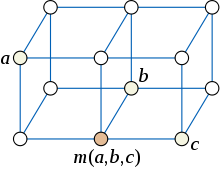The median of three vertices in a median graph

In mathematics, and more specifically graph theory, a median graph is an undirected graph in which any three vertices a, b, and c have a unique median: a vertex m(a,b,c) that belongs to shortest paths between any two of a, b, and c.

The concept of median graphs has long been studied, for instance by Birkhoff & Kiss (1947) or (more explicitly) by Avann (1961), but the first paper to call them "median graphs" appears to be Nebesk'y (1971). As Chung, Graham, and Saks write, "median graphs arise naturally in the study of ordered sets and discrete distributive lattices, and have an extensive literature". In phylogenetics, the Buneman graph representing all maximum parsimony evolutionary trees is a median graph. Median graphs also arise in social choice theory: if a set of alternatives has the structure of a median graph, it is possible to derive in an unambiguous way a majority preference among them.

Additional surveys of median graphs are given by Klavžar & Mulder (1999), Bandelt & Chepoi (2008), and Knuth (2008).

## Examples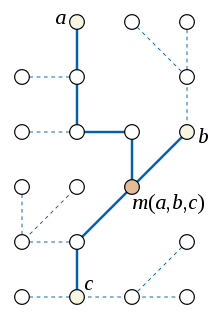The median of three vertices in a tree, showing the subtree formed by the union of shortest paths between the vertices.

Any tree is a median graph. To see this, observe that in a tree, the union of the three shortest paths between any three vertices a, b, and c is either itself a path, or a subtree formed by three paths meeting at a single central node with degree three. If the union of the three paths is itself a path, the median m(a,b,c) is equal to one of a, b, or c, whichever of these three vertices is between the other two in the path. If the subtree formed by the union of the three paths is not a path, the median of the three vertices is the central degree-three node of the subtree.

Additional examples of median graphs are provided by the grid graphs. In a grid graph, the coordinates of the median m(a,b,c) can be found as the median of the coordinates of a, b, and c. Conversely, it turns out that, in any median graph, one may label the vertices by points in an integer lattice in such a way that medians can be calculated coordinatewise in this way.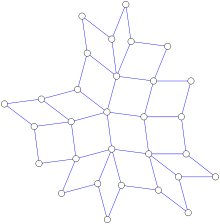A squaregraph.

Squaregraphs, planar graphs in which all interior faces are quadrilaterals and all interior vertices have four or more incident edges, are another subclass of the median graphs. A polyomino is a special case of a squaregraph and therefore also forms a median graph.

The simplex graph κ(G) of any undirected graph G has a node for every clique (complete subgraph) of G; two nodes are linked by an edge if the corresponding cliques differ by one vertex. The median of any three cliques may be formed by using the majority rule to determine which vertices of the cliques to include; the simplex graph is a median graph in which this rule determines the median of any three vertices.

No cycle graph of length other than four can be a median graph, because any such cycle has three vertices a, b, and c such that the three shortest paths wrap all the way around the cycle without having a common intersection. For such a triple of vertices, there can be no median.

## Equivalent definitions

In any graph, for any two vertices a and b, define the interval of vertices that lie on shortest paths

I(a,b) = {v | d(a,b) = d(a,v) + d(v,b)}.

A median graph is defined by the property that, for any three vertices a, b, and c, these intervals intersect in a single point:

For all a, b, and c, |I(a,b) ∩ I(a,c) ∩ I(b,c)| = 1.

Equivalently, for every three vertices a, b, and c one can find a vertex m(a,b,c) such that the unweighted distances in the graph satisfy the equalities

• d(a,b) = d(a,m(a,b,c)) + d(m(a,b,c),b)
• d(a,c) = d(a,m(a,b,c)) + d(m(a,b,c),c)
• d(b,c) = d(b,m(a,b,c)) + d(m(a,b,c),c)

and m(a,b,c) is the only vertex for which this is true.

It is also possible to define median graphs as the solution sets of 2-satisfiability problems, as the retracts of hypercubes, as the graphs of finite median algebras, as the Buneman graphs of Helly split systems, and as the graphs of windex 2; see the sections below.

## Distributive lattices and median algebras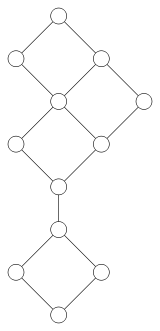The graph of a distributive lattice, drawn as a Hasse diagram.

In lattice theory, the graph of a finite lattice has a vertex for each lattice element and an edge for each pair of elements in the covering relation of the lattice. Lattices are commonly presented visually via Hasse diagrams, which are drawings of graphs of lattices. These graphs, especially in the case of distributive lattices, turn out to be closely related to median graphs.

In a distributive lattice, Birkhoff's self-dual ternary median operation

m(a,b,c) = (ab) ∨ (ac) ∨ (bc) = (ab) ∧ (ac) ∧ (bc),

satisfies certain key axioms, which it shares with the usual median of numbers in the range from 0 to 1 and with median algebras more generally:

The distributive law may be replaced by an associative law:

The median operation may also be used to define a notion of intervals for distributive lattices:

I(a,b) = {x | m(a,x,b) = x} = {x | abxab}.

The graph of a finite distributive lattice has an edge between any two vertices a and b whenever I(a,b) = {a,b}. For any two vertices a and b of this graph, the interval I(a,b) defined in lattice-theoretic terms above consists of the vertices on shortest paths from a to b, and thus coincides with the graph-theoretic intervals defined earlier. For any a, b, and c, m(a,b,c) is the unique intersection of the three intervals I(a,b), I(a,c), and I(b,c). Therefore, the graph of any finite distributive lattice is a median graph. Conversely, if a median graph G contains two vertices 0 and 1 such that every other vertex lies on a shortest path between the two (equivalently, m(0,a,1) = a for all a), then we may define a distributive lattice in which ab = m(a,0,b) and ab = m(a,1,b), and G will be the graph of this lattice.

Duffus & Rival (1983) characterize graphs of distributive lattices directly as diameter-preserving retracts of hypercubes. More generally, any median graph gives rise to a ternary operation m satisfying idempotence, commutativity, and distributivity, but possibly without the identity elements of a distributive lattice. Any ternary operation on a finite set that satisfies these three properties (but that does not necessarily have 0 and 1 elements) gives rise in the same way to a median graph.

## Convex sets and Helly families

In a median graph, a set S of vertices is said to be convex if, for every two vertices a and b belonging to S, the whole interval I(a,b) is a subset of S. Equivalently, given the two definitions of intervals above, S is convex if it contains every shortest path between two of its vertices, or if it contains the median of any three points at least two of which are from S. Observe that the intersection of any two convex sets is itself convex.

The convex sets in a median graph have the Helly property: if F is any family of pairwise-intersecting convex sets, then all sets in F have a common intersection. For, if F has only three convex sets S, T, and U in it, with a in the intersection of the pair S and T, b in the intersection of the pair T and U, and c in the intersection of the pair S and U, then any shortest path from a to b must lie within T by convexity, and similarly any shortest path between the other two pairs of vertices must lie within the other two sets; but m(a,b,c) belongs to paths between all three pairs of vertices, so it lies within all three sets, and forms part of their common intersection. If F has more than three convex sets in it, the result follows by induction on the number of sets, for one may replace any two of the sets in F by their intersection, using the result for triples of sets to show that the replaced family is still pairwise intersecting.

A particularly important family of convex sets in a median graph, playing a role similar to that of halfspaces in Euclidean space, are the sets

Wuv = {w | d(w,u) < d(w,v)}

defined for any edge uv of the graph. In words, Wuv consists of the vertices closer to u than to v, or equivalently the vertices w such that some shortest path from v to w goes through u. To show that Wuv is convex, let w1w2...wk be any shortest path that starts and ends within Wuv; then w2 must also lie within Wuv, for otherwise the two points m1 = m(u,w1,wk) and m2 = m(m1,w2...wk) could be shown (by considering the possible distances between the vertices) to be distinct medians of u, w1, and wk, contradicting the definition of a median graph which requires medians to be unique. Thus, each successive vertex on a shortest path between two vertices of Wuv also lies within Wuv, so Wuv contains all shortest paths between its nodes, one of the definitions of convexity.

The Helly property for the sets Wuv plays a key role in the characterization of median graphs as the solution of 2-satisfiability instances, below.

## 2-satisfiability

Median graphs have a close connection to the solution sets of 2-satisfiability problems that can be used both to characterize these graphs and to relate them to adjacency-preserving maps of hypercubes.

A 2-satisfiability instance consists of a collection of Boolean variables and a collection of clauses, constraints on certain pairs of variables requiring those two variables to avoid certain combinations of values. Usually such problems are expressed in conjunctive normal form, in which each clause is expressed as a disjunction and the whole set of constraints is expressed as a conjunction of clauses, such as$(x_{11} \lor x_{12}) \land (x_{21} \lor x_{22}) \land \cdots \land (x_{n1} \lor x_{n2}) \land \cdots.$

A solution to such an instance is an assignment of truth values to the variables that satisfies all the clauses, or equivalently that causes the conjunctive normal form expression for the instance to become true when the variable values are substituted into it. The family of all solutions has a natural structure as a median algebra, where the median of three solutions is formed by choosing each truth value to be the majority function of the values in the three solutions; it is straightforward to verify that this median solution cannot violate any of the clauses. Thus, these solutions form a median graph, in which the neighbor of any solution is formed by negating a set of variables that are all constrained to be equal or unequal to each other.

Conversely, any median graph G may be represented in this way as the solution set to a 2-satisfiability instance. To find such a representation, create a 2-satisfiability instance in which each variable describes the orientation of one of the edges in the graph (an assignment of a direction to the edge causing the graph to become directed rather than undirected) and each constraint allows two edges to share a pair of orientations only when there exists a vertex v such that both orientations lie along shortest paths from other vertices to v. Each vertex v of G corresponds to a solution to this 2-satisfiability instance in which all edges are directed towards v. Any solution to the instance must come from some vertex v in this way, where v is the common intersection of the sets Wuw for edges directed from w to u; this common intersection exists due to the Helly property of the sets Wuw. Therefore, the solutions to this 2-satisfiability instance correspond one-for-one with the vertices of G.

## Retracts of hypercubes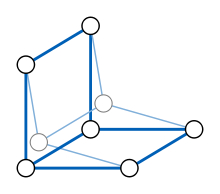Retraction of a cube onto a six-vertex subgraph.

A retraction of a graph G is an adjacency-preserving map from G to one of its subgraphs. More precisely, it is graph homomorphism φ from G to itself such that φ(v) = v for any vertex v in the subgraph φ(G). The image of the retraction is called a retract of G. Retractions are examples of metric maps: the distance between φ(v) and φ(w), for any v and w, is at most equal to the distance between v and w, and is equal whenever v and w both belong to φ(G). Therefore, a retract must be an isometric subgraph of G: distances in the retract equal those in G.

If G is a median graph, and a, b, and c are any three vertices of a retract φ(G), then φ(m(a,b,c)) must be a median of a, b, and c, and so must equal m(a,b,c). Therefore, φ(G) contains medians of any triples of its vertices, and must also be a median graph. In other words, the family of median graphs is closed under the retraction operation.

A hypercube graph, in which the vertices correspond to all possible k-bit bitvectors and in which two vertices are adjacent when the corresponding bitvectors differ in only a single bit, is a special case of a k-dimensional grid graph and is therefore a median graph. The median of any three bitvectors a, b, and c may be calculated by computing, in each bit position, the majority function of the bits of a, b, and c. Since median graphs are closed under retraction, and include the hypercubes, every retract of a hypercube is a median graph.

Conversely, every median graph must be the retract of a hypercube. This may be seen from the connection, described above, between median graphs and 2-satisfiability: let G be the graph of solutions to a 2-satisfiability instance; without loss of generality this instance can be formulated in such a way that no two variables are always equal or always unequal in every solution. Then the space of all truth assignments to the variables of this instance forms a hypercube. For each clause, formed as the disjunction of two variables or their complements, in the 2-satisfiability instance, one can form a retraction of the hypercube in which truth assignments violating this clause are mapped to truth assignments in which both variables satisfy the clause, without changing the other variables in the truth assignment. The composition of the retractions formed in this way for each of the clauses gives a retraction of the hypercube onto the solution space of the instance, and therefore gives a representation of G as the retract of a hypercube. In particular, median graphs are isometric subgraphs of hypercubes, and are therefore partial cubes. However, not all partial cubes are median graphs; for instance, a six-vertex cycle graph is a partial cube but is not a median graph.

As Imrich & Klavžar (2000) describe, an isometric embedding of a median graph into a hypercube may be constructed in time O(m log n), where n and m are the numbers of vertices and edges of the graph respectively.

## Triangle-free graphs and recognition algorithms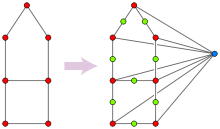Converting a triangle-free graph into a median graph.

The problems of testing whether a graph is a median graph, and whether a graph is triangle-free, both had been well studied when Imrich, Klavžar & Mulder (1999) observed that, in some sense, they are computationally equivalent. Therefore, the best known time bound for testing whether a graph is triangle-free, O(m1.41), applies as well to testing whether a graph is a median graph, and any improvement in median graph testing algorithms would also lead to an improvement in algorithms for detecting triangles in graphs.

In one direction, suppose one is given as input a graph G, and must test whether G is triangle-free. From G, construct a new graph H having as vertices each set of zero, one, or two adjacent vertices of G. Two such sets are adjacent in H when they differ by exactly one vertex. An equivalent description of H is that it is formed by splitting each edge of G into a path of two edges, and adding a new vertex connected to all the original vertices of G. This graph H is by construction a partial cube, but it is a median graph only when G is triangle-free: if a, b, and c form a triangle in G, then {a,b}, {a,c}, and {b,c} have no median in H, for such a median would have to correspond to the set {a,b,c}, but sets of three or more vertices of G do not form vertices in H. Therefore, G is triangle-free if and only if H is a median graph. In the case that G is triangle-free, H is its simplex graph. An algorithm to test efficiently whether H is a median graph could by this construction also be used to test whether G is triangle-free. This transformation preserves the computational complexity of the problem, for the size of H is proportional to that of G.

The reduction in the other direction, from triangle detection to median graph testing, is more involved and depends on the previous median graph recognition algorithm of Hagauer, Imrich & Klavžar (1999), which tests several necessary conditions for median graphs in near-linear time. The key new step involves using a breadth first search to partition the graph into levels according to their distances from some arbitrarily chosen root vertex, forming a graph in each level in which two vertices are adjacent if they share a common neighbor in the previous level, and searching for triangles in these graphs. The median of any such triangle must be a common neighbor of the three triangle vertices; if this common neighbor does not exist, the graph is not a median graph. If all triangles found in this way have medians, and the previous algorithm finds that the graph satisfies all the other conditions for being a median graph, then it must actually be a median graph. Note that this algorithm requires, not just the ability to test whether a triangle exists, but a list of all triangles in the level graph. In arbitrary graphs, listing all triangles sometimes requires Ω(m3/2) time, as some graphs have that many triangles, however Hagauer et al. show that the number of triangles arising in the level graphs of their reduction is near-linear, allowing the Alon et al. fast matrix multiplication based technique for finding triangles to be used.

## Evolutionary trees, Buneman graphs, and Helly split systems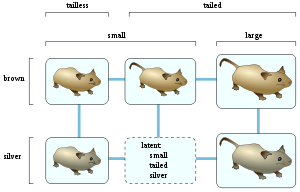The Buneman graph for five types of mouse.

Phylogeny is the inference of evolutionary trees from observed characteristics of species; such a tree must place the species at distinct vertices, and may have additional latent vertices, but the latent vertices are required to have three or more incident edges and must also be labeled with characteristics. A characteristic is binary when it has only two possible values, and a set of species and their characteristics exhibit perfect phylogeny when there exists an evolutionary tree in which the vertices (species and latent vertices) labeled with any particular characteristic value form a contiguous subtree. If a tree with perfect phylogeny is not possible, it is often desired to find one exhibiting maximum parsimony, or equivalently, minimizing the number of times the endpoints of a tree edge have different values for one of the characteristics, summed over all edges and all characteristics.

Buneman (1971) described a method for inferring perfect phylogenies for binary characteristics, when they exist. His method generalizes naturally to the construction of a median graph for any set of species and binary characteristics, which has been called the median network or Buneman graph and is a type of phylogenetic networks. Any maximum parsimony evolutionary tree embeds into the Buneman graph, in the sense that tree edges follow paths in the graph and the number of characteristic value changes on the tree edge is the same as the number in the corresponding path. The Buneman graph will be a tree if and only if a perfect phylogeny exists; this happens when there are no two incompatible characteristics for which all four combinations of characteristic values are observed.

To form the Buneman graph for a set of species and characteristics, first, eliminate redundant species that are indistinguishable from some other species and redundant characteristics that are always the same as some other characteristic. Then, form a latent vertex for every combination of characteristic values such that every two of the values exist in some known species. In the example shown, there are small brown tailless mice, small silver tailless mice, small brown tailed mice, large brown tailed mice, and large silver tailed mice; the Buneman graph method would form a latent vertex corresponding to an unknown species of small silver tailed mice, because every pairwise combination (small and silver, small and tailed, and silver and tailed) is observed in some other known species. However, the method would not infer the existence of large brown tailless mice, because no mice are known to have both the large and tailless traits. Once the latent vertices are determined, form an edge between every pair of species or latent vertices that differ in a single characteristic.

One can equivalently describe a collection of binary characteristics as a split system, a family of sets having the property that the complement set of any set in the family is also in the family. This split system has a set for each characteristic value, consisting of the species that have that value. When the latent vertices are included, the resulting split system has the Helly property: any pairwise intersecting family of sets has a common intersection. In some sense median graphs are characterized as coming from Helly split systems: the pairs (Wuv, Wvu) defined for each edge uv of a median graph form a Helly split system, so if one applies the Buneman graph construction to this system no latent vertices will be needed and the result will be the same as the starting graph.

Bandelt et al. (1995) and Bandelt, Macaulay & Richards (2000) describe techniques for simplified hand calculation of the Buneman graph, and use this construction to visualize human genetic relationships.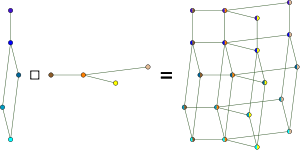The Cartesian product of graphs forms a median graph from two smaller median graphs.
• The Cartesian product of any two median graphs is another median graph. Medians in the product graph may be computed by independently finding the medians in the two factors, just as medians in grid graphs may be computed by independently finding the median in each linear dimension.
• The windex of a graph measures the amount of lookahead needed to optimally solve a problem in which one is given a sequence of graph vertices si, and must find as output another sequence of vertices ti minimizing the sum of the distances d(si,ti) and d(ti − 1,ti). Median graphs are exactly the graphs that have windex 2. In a median graph, the optimal choice is to set ti = m(ti − 1,si,si + 1).
• The property of having a unique median is also called the unique Steiner point property. An optimal Steiner tree for any three vertices a, b, and c in a median graph may be found as the union of three shortest paths, from a, b, and c to m(a,b,c). Bandelt & Barthélémy (1984) study more generally the problem of finding the vertex minimizing the sum of distances to each of a given set of vertices, and show that it has a unique solution for any odd number of vertices in a median graph. They also show that this median of a set S of vertices in a median graph satisfies the Condorcet criterion for the winner of an election: compared to any other vertex, it is closer to a majority of the vertices in S.
• As with partial cubes more generally, any median graph with n vertices has at most (n/2) log2 n edges. However, the number of edges cannot be too small: Klavžar, Mulder & Škrekovski (1998) prove that in any median graph the inequality 2n − m − k ≤ 2 holds, where m is the number of edges and k is the dimension of the hypercube that the graph is a retract of. This inequality is an equality if and only if the median graph contains no cubes. This is a consequence of another identity for median graphs: the Euler characteristic Σ (-1)dim(Q) is always equal to one, where the sum is taken over all hypercube subgraphs Q of the given median graph.
• The only regular median graphs are the hypercubes.

Wikimedia Foundation. 2010.

### Look at other dictionaries:

• Median algebra — In mathematics, a median algebra is a set with a ternary operation < x,y,z > satisfying a set of axioms which generalise the notion of median or majority function, as a Boolean function. The axioms are < x,y,y > = y < x,y,z > =… …   Wikipedia

• Median — This article is about the statistical concept. For other uses, see Median (disambiguation). In probability theory and statistics, a median is described as the numerical value separating the higher half of a sample, a population, or a probability… …   Wikipedia

• Graph homomorphism — Not to be confused with graph homeomorphism. In the mathematical field of graph theory a graph homomorphism is a mapping between two graphs that respects their structure. More concretely it maps adjacent vertices to adjacent vertices. Contents 1… …   Wikipedia

• graph — /graf, grahf/, n. 1. a diagram representing a system of connections or interrelations among two or more things by a number of distinctive dots, lines, bars, etc. 2. Math. a. a series of points, discrete or continuous, as in forming a curve or… …   Universalium

• Clique (graph theory) — A graph with 23 1 vertex cliques (its vertices), 42 2 vertex cliques (its edges), 19 3 vertex cliques (the light blue triangles), and 2 4 vertex cliques (dark blue). Six of the edges and 11 of the triangles form maximal cliques. The two dark blue …   Wikipedia

• Triangle-free graph — In the mathematical area of graph theory, a triangle free graph is an undirected graph in which no three vertices form a triangle of edges. Triangle free graphs may be equivalently defined as graphs with clique number ≤ 2, graphs with girth ≥ 4,… …   Wikipedia

• Hypercube graph — The hypercube graph Q4 Vertices 2n Edges 2n−1n …   Wikipedia

• Lattice graph — The terms lattice graph, mesh graph, or grid graph refer to a number of categories of graphs whose drawing corresponds to some grid/mesh/lattice, i.e., its vertices correspond to the nodes of the mesh and its edges correspond to the ties between… …   Wikipedia

• Geometric graph theory — In mathematics, a geometric graph is a graph in which the vertices or edges are associated with geometric objects or configurations. Geometric graph theory is a specialization of graph theory that studies geometric graphs. Notable geometric… …   Wikipedia

• Tree (graph theory) — Trees A labeled tree with 6 vertices and 5 edges Vertices v Edges v 1 Chromatic number …   Wikipedia